## LeNet-5 - A Classic CNN ArchitectureArticle originally posted on Data Science Central. Visit Data Science Central

Yann LeCun, Leon Bottou, Yosuha Bengio and Patrick Haffner proposed a neural network architecture for handwritten and machine-printed character recognition in 1990’s which they called LeNet-5. The architecture is straightforward and simple to understand that’s why it is mostly used as a first step for teaching Convolutional Neural Network.

### LeNet-5 Architecture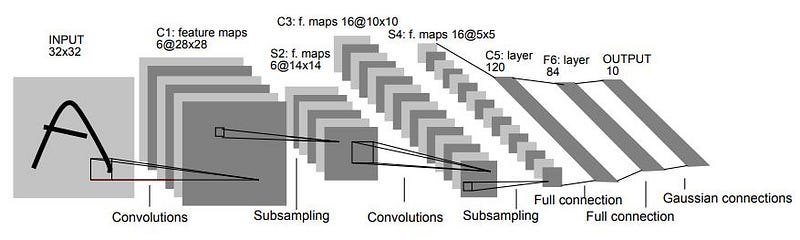Original image published in [LeCun et al., 1998]

The LeNet-5 architecture consists of two sets of convolutional and average pooling layers, followed by a flattening convolutional layer, then two fully-connected layers and finally a softmax classifier.

First Layer:

The input for LeNet-5 is a 32×32 grayscale image which passes through the first convolutional layer with 6 feature maps or filters having size 5×5 and a stride of one. The image dimensions changes from 32x32x1 to 28x28x6.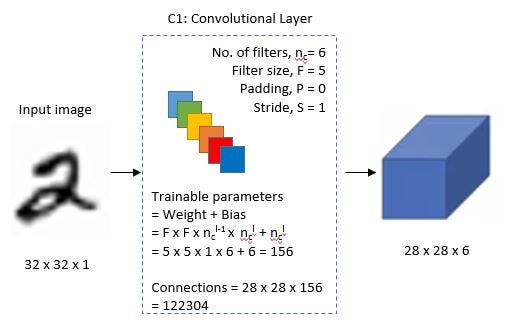Second Layer:

Then the LeNet-5 applies average pooling layer or sub-sampling layer with a filter size 2×2 and a stride of two. The resulting image dimensions will be reduced to 14x14x6.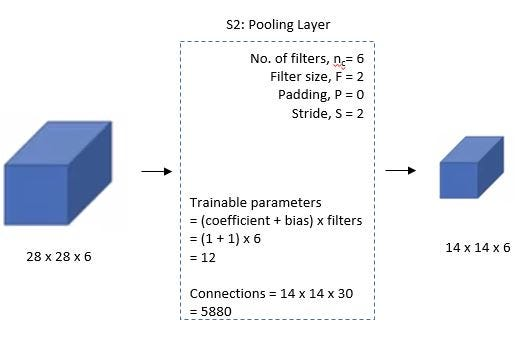Third Layer:

Next, there is a second convolutional layer with 16 feature maps having size 5×5 and a stride of 1. In this layer, only 10 out of 16 feature maps are connected to 6 feature maps of the previous layer as shown below.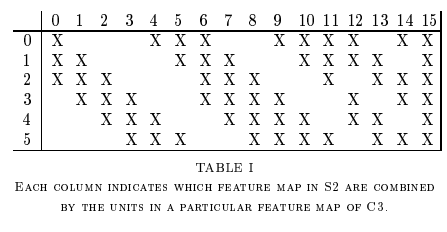The main reason is to break the symmetry in the network and keeps the number of connections within reasonable bounds. That’s why the number of training parameters in this layers are 1516 instead of 2400 and similarly, the number of connections are 151600 instead of 240000.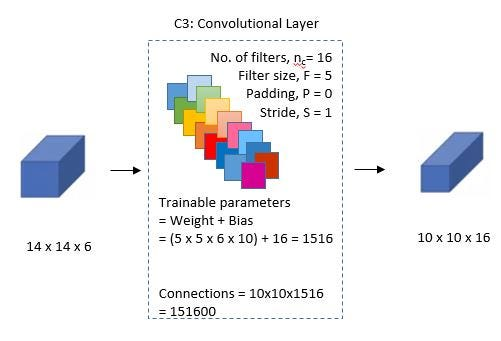Fourth Layer:

The fourth layer (S4) is again an average pooling layer with filter size 2×2 and a stride of 2. This layer is the same as the second layer (S2) except it has 16 feature maps so the output will be reduced to 5x5x16.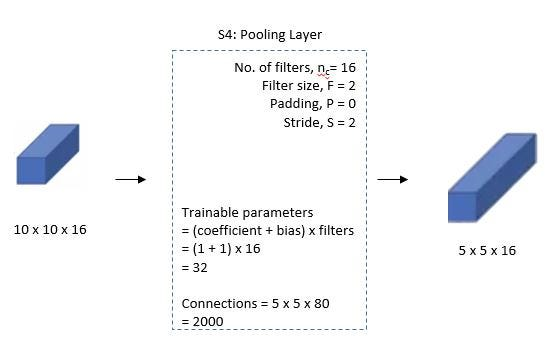Fifth Layer:

The fifth layer (C5) is a fully connected convolutional layer with 120 feature maps each of size 1×1. Each of the 120 units in C5 is connected to all the 400 nodes (5x5x16) in the fourth layer S4.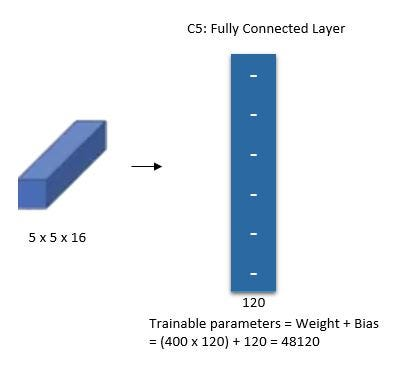Sixth Layer:

The sixth layer is a fully connected layer (F6) with 84 units.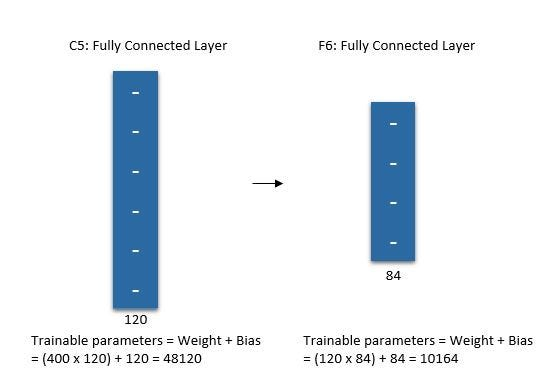Output Layer:

Finally, there is a fully connected softmax output layer ŷ with 10 possible values corresponding to the digits from 0 to 9.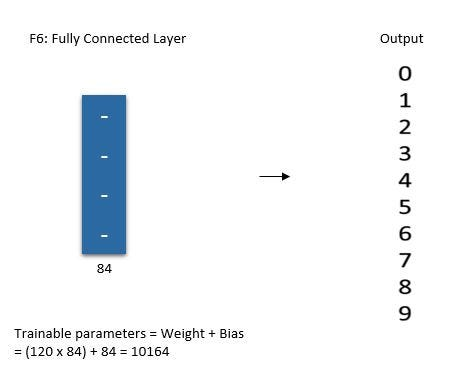#### Summary of LeNet-5 Architecture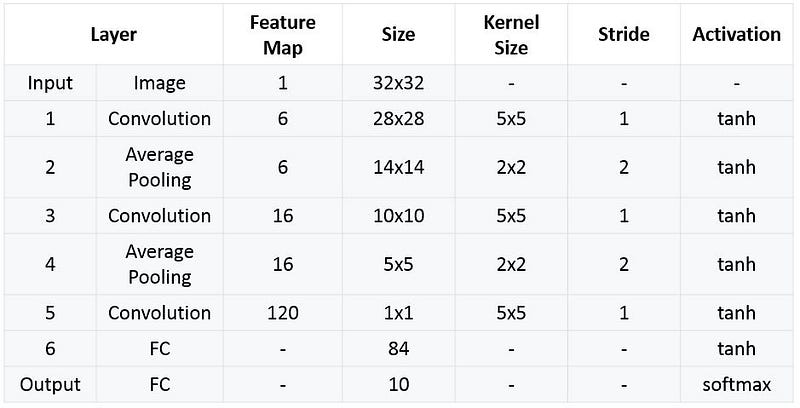LeNet-5 Architecture Summarized Table

### Implementation of LeNet-5 Using Keras

We will download the MNIST dataset under the Keras API and normalize it as we did in the earlier post.

For details, please visit: Implementation of CNN using Keras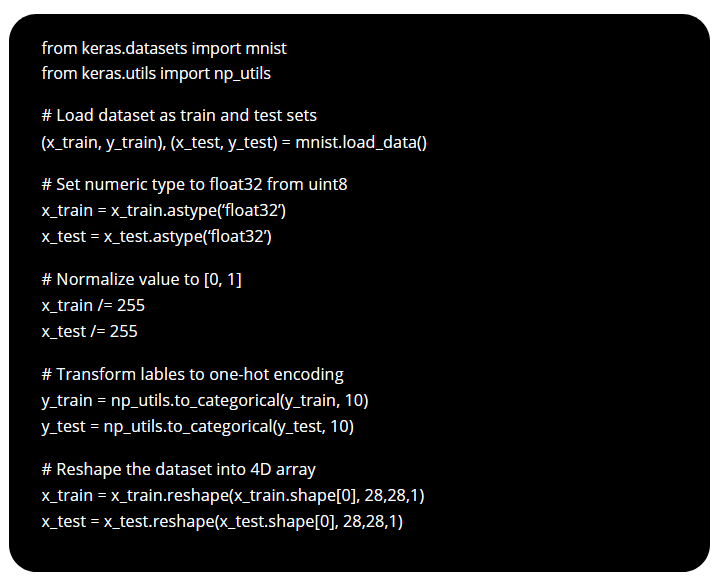#### Define LeNet-5 Model

Create a new instance of a model object using sequential model API. Then add layers to the neural network as per LeNet-5 architecture discussed earlier. Finally, compile the model with the ‘categorical_crossentropy’ loss function and ‘SGD’ cost optimization algorithm. When compiling the model, add metrics=[‘accuracy’] as one of the parameters to calculate the accuracy of the model.

It is important to highlight that each image in the MNIST data set has a size of 28 X 28 pixels so we will use the same dimensions for LeNet-5 input instead of 32 X 32 pixels.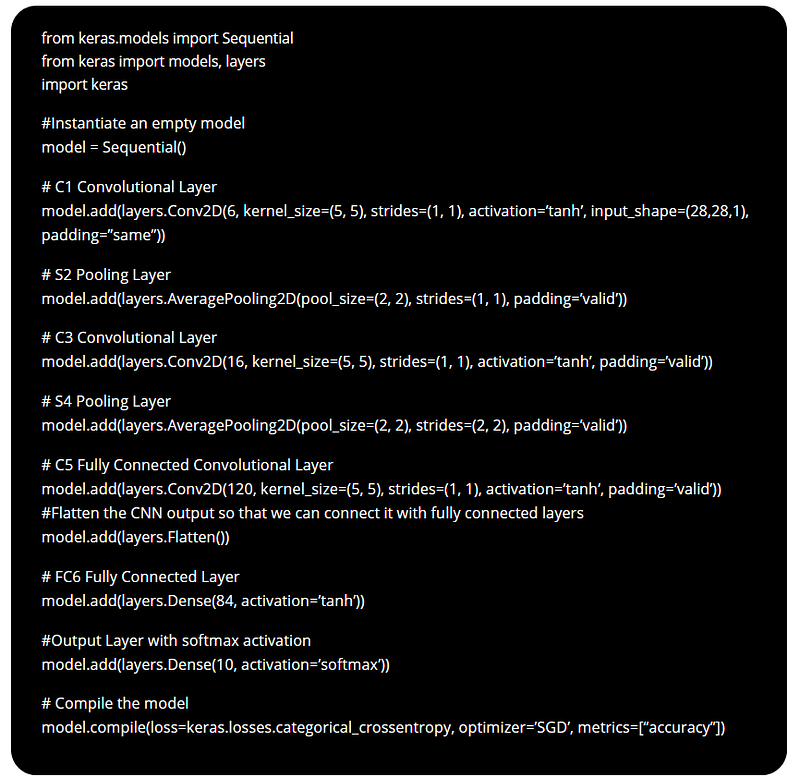We can train the model by calling model.fit function and pass in the training data, the expected output, number of epochs, and batch size. Additionally, Keras provides a facility to evaluate the loss and accuracy at the end of each epoch. For the purpose, we can split the training data using ‘validation_split’ argument or use another dataset using ‘validation_data’ argument. We will use our training dataset to evaluate the loss and accuracy after every epoch.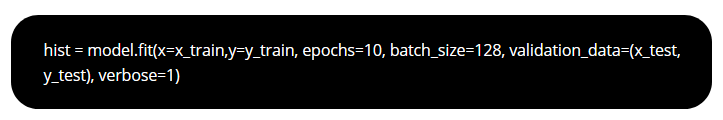#### Evaluate the Model

We can test the model by calling model.evaluate and passing in the testing data set and the expected output.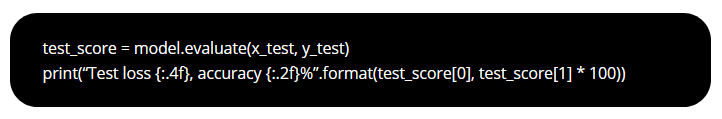#### Visualize the Training Process

We will visualize the training process by plotting the training accuracy and loss after each epoch.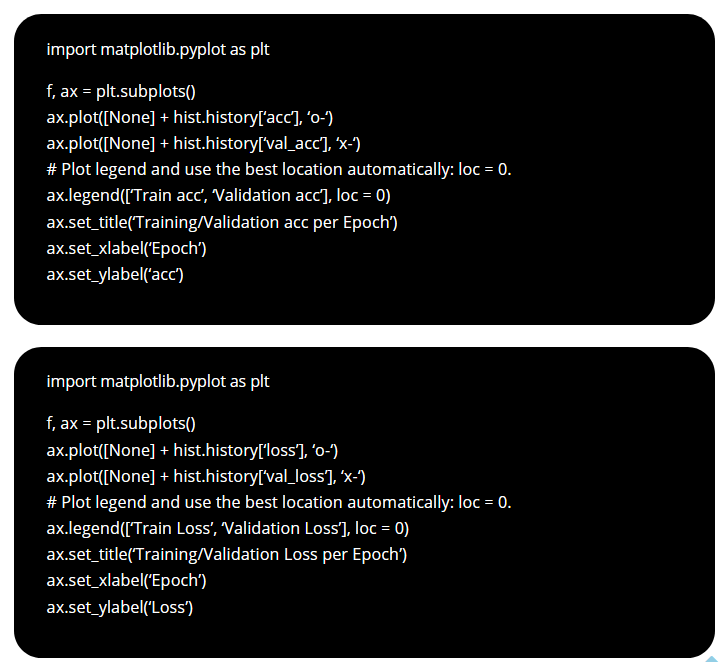### Summary

• We understood the LeNet-5 architecture in details.
• We learned the implementation of LeNet-5 using Keras.